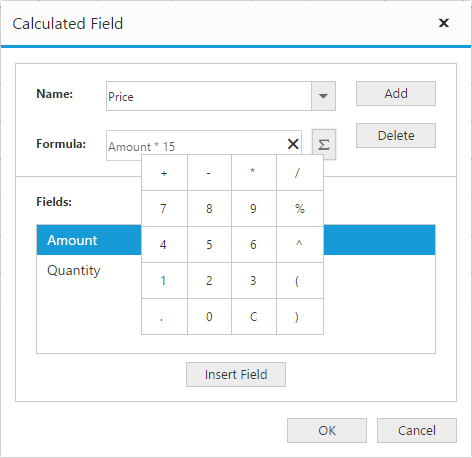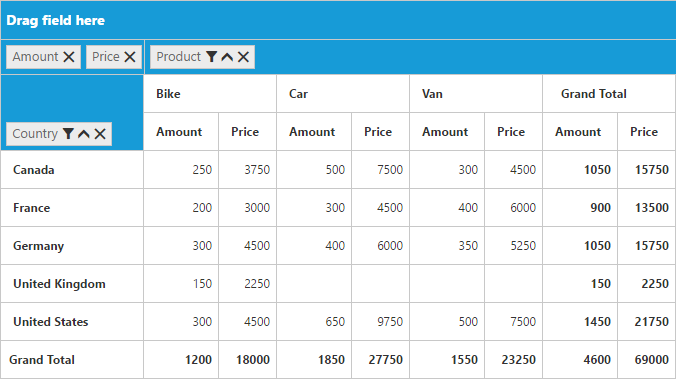# Calculated Field

27 Feb 20182 minutes to read

NOTE

This feature is applicable only for the relational data source.

The pivot grid provides support to insert a new calculated field based on the existing pivot fields through the calculated field dialog or code behind.

### Through UI

To insert a new calculated field, open the calculated field dialog by using the grouping bar context menu. You can define “Name” for the new calculated field, and “Formula” can be entered by inserting required fields through the fields section. For inserting numbers and operators, you can use the formula pop-up as shown in the below screen-shot:Click Add to add the respective calculated field, and then click OK to populate the pivot grid control.

### Through code-behind

For client mode, the calculated field can be created at code-behind by defining formula based on the existing pivot fields in the pivot grid. To indicate a field as a calculated field, you should set the `isCalculatedField` property to true and `formula` property to set the expression.

• HTML
• ``````<template>
<div>
<ej-pivot-grid id="groupingBar" e-data-source.bind="pivotData" e-enable-grouping-bar="true" e-is-calculated-field="true">
</ej-pivot-grid>
</div>
</template>``````
• JS
• ``````export class BasicUse {
constructor (){
this.pivotData: {
//...
values: [
{
fieldName: "Amount",
fieldCaption: "Amount"
},
{
fieldName: "Price",
fieldCaption: "Price",
isCalculatedField: true,
formula: "Amount*15"
}
]
},
}
}``````